Warning

This documents an unmaintained version of NetworkX. Please upgrade to a maintained version and see the current NetworkX documentation.

# power¶

power(G, k)[source]

Returns the specified power of a graph.

The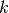-th power of a simple graph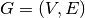is the graph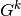whose vertex set is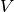, two distinct vertices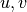are adjacent inif and only if the shortest path distance between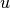and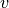in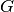is at most.

Parameters: G (graph) – A NetworkX simple graph object. k (positive integer) – The power to which to raise the graph.to the-th power. NetworkX simple graph exc: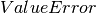– If the exponentis not positive. NetworkXError – If G is not a simple graph.

Examples

>>> G = nx.path_graph(4)
>>> nx.power(G,2).edges()
[(0, 1), (0, 2), (1, 2), (1, 3), (2, 3)]
>>> nx.power(G,3).edges()
[(0, 1), (0, 2), (0, 3), (1, 2), (1, 3), (2, 3)]


A complete graph of order n is returned if k is greater than equal to n/2 for a cycle graph of even order n, and if k is greater than equal to (n-1)/2 for a cycle graph of odd order.

>>> G = nx.cycle_graph(5)
>>> nx.power(G,2).edges() == nx.complete_graph(5).edges()
True
>>> G = nx.cycle_graph(8)
>>> nx.power(G,4).edges() == nx.complete_graph(8).edges()
True


References

  Bondy, U. S. R. Murty, Graph Theory. Springer, 2008.

Notes

Exercise 3.1.6 of Graph Theory by J. A. Bondy and U. S. R. Murty .